# Refraction

Definition: Refraction

Refraction is the bending of light as it passes between materials of different optical density.

Definition: Index of Refraction

Index of Refraction of a material is the ratio of the speed of light in vacuum to the speed of light in that material:

 n =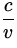(1)

where v is the speed of light in the material.

Note:

• The more dense the material, the slower the speed of light in that material. Thus n > 1 for all materials, and increases with increasing density. n = 1 in vacuum.
• The frequency of light does not change when it passes from one medium to another. According to the formula v =f , the wavelength must change. The index of refraction can therefore be written in terms of wavelengths as:

 n =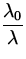(2)

where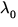is the wavelength of the light in the vacuum andis the wavelength of the light in the medium.

Idea: Explanation for Refraction of Light

The change in speed and wavelength at the boundary between two materials causes light to change direction. Think of a car approaching a patch of mud at a sharp angle from a well paved road. The tire that hits the mud first will slow down, while the other tire is still going fast on the good road. This will cause the car to turn, until both tires are in the mud and going at the same speed. If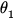is the angle of the ray relative to the normal to the surface in medium 1, and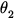is the angle relative to the normal in medium 2, then: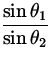==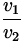=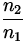(3)

where v1 andare the speed and wavelength in medium 1, etc. This is illustrated in Fig. 22.6.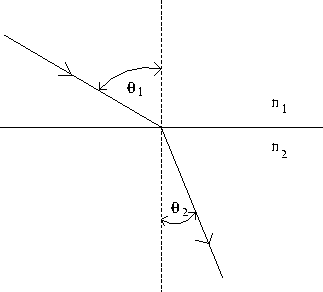Note:

• This relationship between the angles is called Snell's Law.
• The relation between the two angles is the same whether the ray is moving from medium 1 to 2 (so thatis the angle of incidence andis the angle of refraction) or whether the ray moves from medium 2 to medium 1, so thatis the angle of incidence andis the angle of refraction.
Total Internal Reflection

For a light ray passing from a more dense to a less dense material, there is a critical angle of incidencefor which the angle of refraction is 90 o . For greater angles of incidence, the light cannot pass through the boundary between the materials, and is reflected within the more dense material. For a light ray trying to pass from medium 2 to medium 1, the critical angle is given by:

sin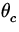=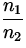sin 90 o =where n1 is the index of refraction of the less dense material, and n2 is the index of refraction of the more dense material.

Note: The formula for the critical angle shows that n2 must be greater than n1 for there to be total internal reflection. That is, medium 2 must be more dense than medium 1. Otherwise sin> 1 , which is not possible.Next: Dispersion Up: Wave Properties of Light Previous: Reflection